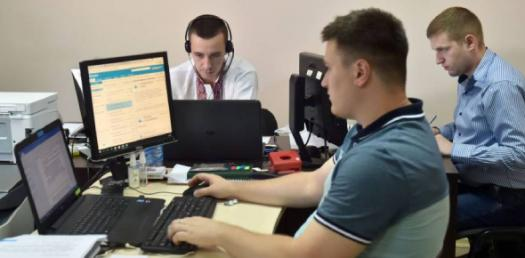# How A Computer Works? Trivia Quiz!

25 Questions | Total Attempts: 5330SettingsThe computer has made our life easier when preparing documents, projects, presentations, doing calculations. Since the advent of computer technology, people have upgraded their skills and work smartly. But, how many of you know about hardware software and programming? Seems very few. Take this quiz to test your knowledge about the technical aspect of the computer.

• 1.
There are _____ main types of memory.
• A.

1

• B.

2

• C.

4

• D.

6

• 2.
An example of an input device is:
• A.

Keyboard

• B.

Monitor

• C.

Speakers

• D.

Printer

• 3.
Computers only function by doing _______________.
• A.

• B.

Writing

• C.

Math

• D.

Science

• 4.
The microprocessor is the main part of the computer?
• A.

True

• B.

False

• 5.
RAM is a type of ____________________________.
• A.

Input

• B.

Output

• C.

Microprocessor

• D.

Memory

• 6.
Computers think like a human brain.
• A.

True

• B.

False

• 7.
An example of an output device is:
• A.

Keyboard

• B.

Mouse

• C.

Printer

• D.

Scanner

• 8.
____________________ tells the microprocessor what to do.
• A.

Output

• B.

Software

• C.

Little humans inside the CPU

• D.

Gate

• 9.
Computers do math very _________________
• A.

Slow

• B.

Fast

• 10.
The first step of a microprocessor is ______________.
• A.

Decode

• B.

Execute

• C.

Fetch

• D.

Crash

• 11.
The second step of a microprocessor is _____________________.
• A.

Fetch

• B.

Execute

• C.

Power

• D.

Decode

• 12.
The third step of a microprocessor is ____________________.
• A.

Execute

• B.

Fetch

• C.

Crash

• D.

Decode

• 13.
A microprocessor works millions of times per _________________.
• A.

Minute

• B.

Hour

• C.

Second

• D.

Day

• 14.
A binary code is made up of ___________________.
• A.

1s and 2s

• B.

0s and 2s

• C.

1s and 4s

• D.

0s and 1s

• 15.
Decode decides on the ______________
• A.

Math operation

• B.

Spelling

• C.

Software

• D.

Output device

• 16.
Fetch receives the data from the memory/software.
• A.

True

• B.

False

• 17.
What does execute do in the microprocessor?
• A.

Fetches data from software

• B.

Decides on the math operation

• C.

Does the math

• D.

Burps

• 18.
There are millions of tiny _________________ on a microprocessor.
• A.

Hairs

• B.

Transistors

• C.

Holes

• D.

Scanners

• 19.
A _________ is the smallest unit of digital information.
• A.

Pixel

• B.

Bit

• C.

Byte

• D.

Centimeter

• 20.
The smallest part of a digital picture is called a ___________.
• A.

Byte

• B.

Gigabyte

• C.

Bit

• D.

Pixel

• 21.
A transistor is made of _________________, a semiconductor.
• A.

Gate

• B.

Silicon

• C.

Source

• D.

Drain

• 22.
Electrons in a  transistor "jump" from the source to the ______________.
• A.

Gate

• B.

Semiconductor

• C.

Drain

• D.

Software

• 23.
Microprocessors are made in a ______________.
• A.

Lab

• B.

Factory

• C.

Fab

• D.

House

• 24.
A fab must be very _______________ to protect the delicate circuits of a microprocessor.
• A.

Bright

• B.

Sterile

• C.

Secure

• D.

Secret

• 25.
There are _______ steps of a microprocessor.
• A.

Two

• B.

Three

• C.

Four

• D.

Five

Related TopicsBack to top